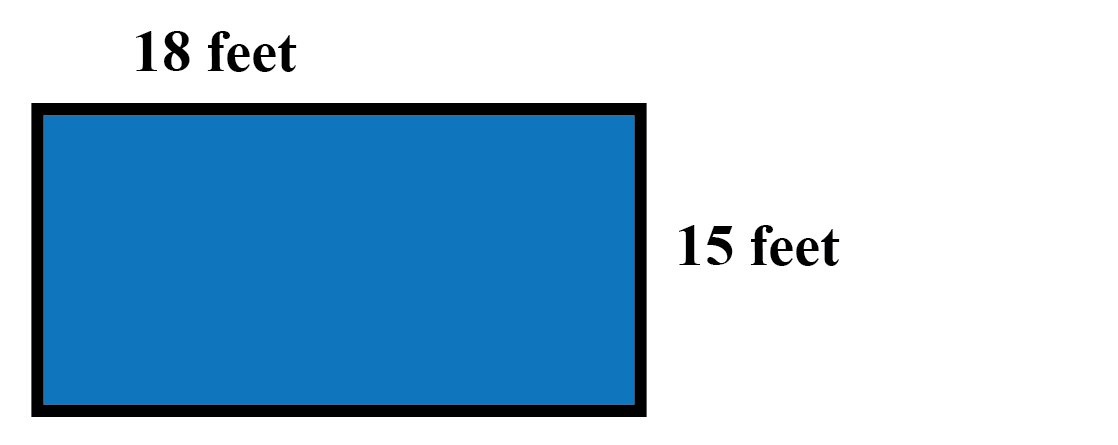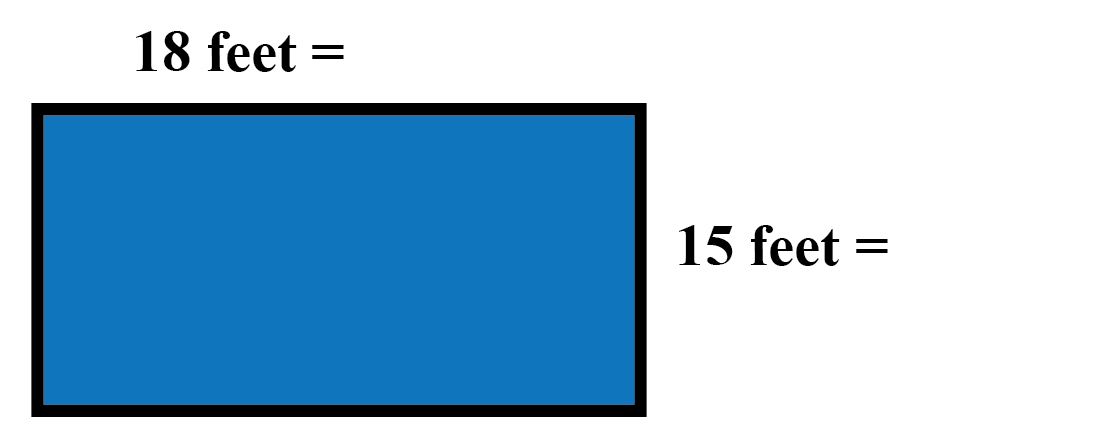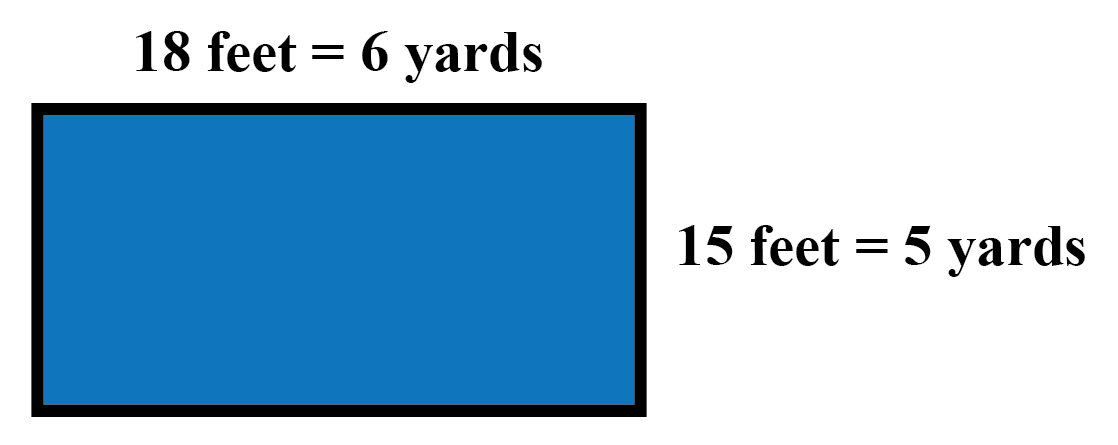Home > ACC6 > Chapter cc24 > Lesson cc24.1.1 > Problem4-8

4-8.

Tina’s rectangular living-room floor measures $15$ feet by $18$ feet. Homework Help ✎1. How many square feet of carpet will Tina need to cover the entire floor?

If it helps, draw a diagram. Find the area of the rectangle that represents the floor.

270 square feet

2. The carpet Tina likes is sold by the square yard. How many square yards will she need? ($1 \text{ yard}=3\text{ feet}$)

There are two ways to solve part (b). You can take your answer from part (a) and divide it by the number of square feet
in a square yard, or you can convert the dimensions of the room into yards and then multiply.

If you use the first method, remember to convert your units properly. A square yard is $3$ feet by $3$ feet, or $9$ square feet.